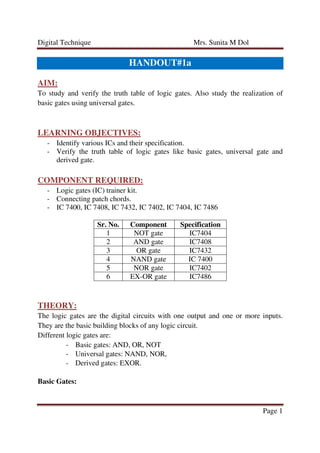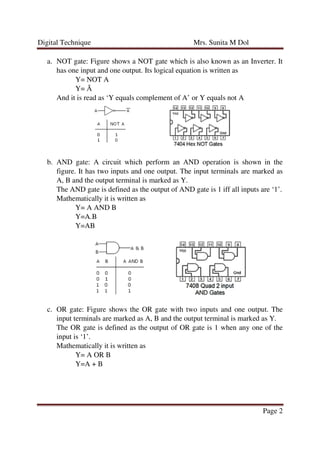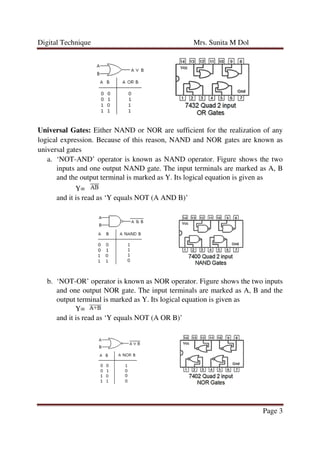Ce diaporama a bien été signalé.
Le téléchargement de votre SlideShare est en cours. ×
Publicité
Publicité
Publicité
Publicité
Publicité
Publicité
Publicité
Publicité
Publicité
Publicité
PublicitéPublicité
Chargement dans…3
×

## Consultez-les par la suite

1 sur 5 Publicité

# Assignment#1a

To study and verify the truth table of logic gates. Also study the realization of
basic gates using universal gates.

To study and verify the truth table of logic gates. Also study the realization of
basic gates using universal gates.

Publicité
Publicité

### Assignment#1a

1. 1. Digital Technique Mrs. Sunita M Dol Page 1 HANDOUT#1a AIM: To study and verify the truth table of logic gates. Also study the realization of basic gates using universal gates. LEARNING OBJECTIVES: - Identify various ICs and their specification. - Verify the truth table of logic gates like basic gates, universal gate and derived gate. COMPONENT REQUIRED: - Logic gates (IC) trainer kit. - Connecting patch chords. - IC 7400, IC 7408, IC 7432, IC 7402, IC 7404, IC 7486 Sr. No. Component Specification 1 NOT gate IC7404 2 AND gate IC7408 3 OR gate IC7432 4 NAND gate IC 7400 5 NOR gate IC7402 6 EX-OR gate IC7486 THEORY: The logic gates are the digital circuits with one output and one or more inputs. They are the basic building blocks of any logic circuit. Different logic gates are: - Basic gates: AND, OR, NOT - Universal gates: NAND, NOR, - Derived gates: EXOR. Basic Gates:
2. 2. Digital Technique Mrs. Sunita M Dol Page 2 a. NOT gate: Figure shows a NOT gate which is also known as an Inverter. It has one input and one output. Its logical equation is written as Y= NOT A Y= Ā And it is read as ‘Y equals complement of A’ or Y equals not A b. AND gate: A circuit which perform an AND operation is shown in the figure. It has two inputs and one output. The input terminals are marked as A, B and the output terminal is marked as Y. The AND gate is defined as the output of AND gate is 1 iff all inputs are ‘1’. Mathematically it is written as Y= A AND B Y=A.B Y=AB c. OR gate: Figure shows the OR gate with two inputs and one output. The input terminals are marked as A, B and the output terminal is marked as Y. The OR gate is defined as the output of OR gate is 1 when any one of the input is ‘1’. Mathematically it is written as Y= A OR B Y=A + B
3. 3. Digital Technique Mrs. Sunita M Dol Page 3 Universal Gates: Either NAND or NOR are sufficient for the realization of any logical expression. Because of this reason, NAND and NOR gates are known as universal gates a. ‘NOT-AND’ operator is known as NAND operator. Figure shows the two inputs and one output NAND gate. The input terminals are marked as A, B and the output terminal is marked as Y. Its logical equation is given as Y= and it is read as ‘Y equals NOT (A AND B)’ b. ‘NOT-OR’ operator is known as NOR operator. Figure shows the two inputs and one output NOR gate. The input terminals are marked as A, B and the output terminal is marked as Y. Its logical equation is given as Y= and it is read as ‘Y equals NOT (A OR B)’
4. 4. Digital Technique Mrs. Sunita M Dol Page 4 Derived gates: a. It is bot basic gate and can be performed using basic gates AND, OR, NOT or Universal gate NAND or NOR. Its logic equation can be written as Y= A EX-OR B When both inputs are same then output is zero whereas the inputs are not same, the output is Basic gates using Univeral gates The three basic operation using NAND and NOR is shown in the following figure. Using NAND gate Using NOR gate
5. 5. Digital Technique Mrs. Sunita M Dol Page 5 PROCEDURE: 1. Check the components for their working. 2. Insert the appropriate IC into the IC base. 3. Make connections as shown in the circuit diagram. 4. Provide the input data via the input switches and observe the output on output LEDs 5. Give various combinations of inputs and note down the output with help of LED for all gate ICs one by one. RESULT: Thus we have verified the truth table of following logic gates: - Basic gates: AND, OR, NOT - Universal gates: NAND, NOR, - Derived gates: EXOR. The realization of basic gates AND, OR and NOT using universal gates NAND and NOR gate is also verified.